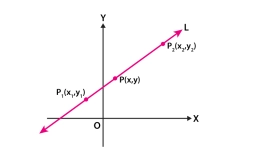# Michael 6

Michael was assigned math problems to complete for his weekly homework. So far, he has completed 28 problems, which is 2/7 of the total number of math problems he was assigned. How many total math problems was Michael assigned for homework?

n =  98

### Step-by-step explanation:Did you find an error or inaccuracy? Feel free to write us. Thank you!

Tips for related online calculators
Need help calculating sum, simplifying, or multiplying fractions? Try our fraction calculator.
Do you have a linear equation or system of equations and looking for its solution? Or do you have a quadratic equation?